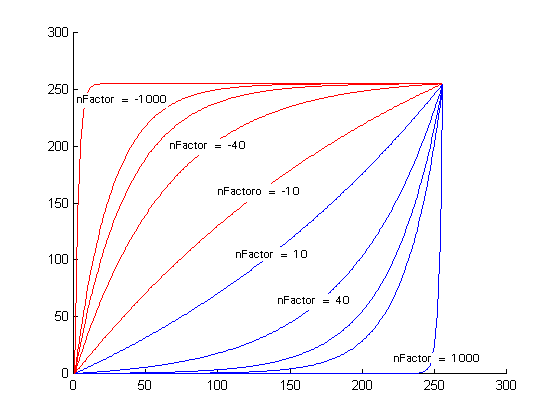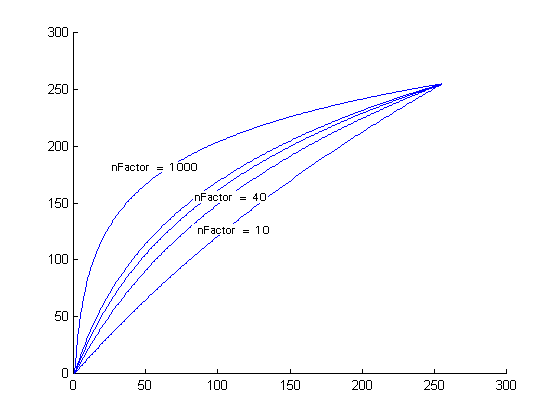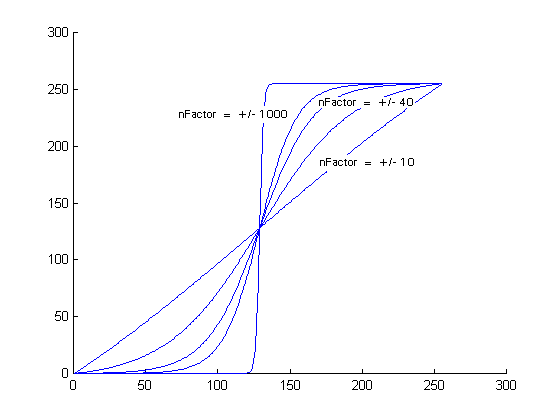﻿ GetFunctionalLookupTable Method (EffectsUtilities) | Leadtools.ImageProcessing.Effects | Raster, Medical, Document Help
←Select platform
In This Topic ▼

# GetFunctionalLookupTable Method

Summary
Updates a range of entries in the lookup table, based on the specified mathematical function.
Syntax
C#
Objective-C
C++/CLI
Java
Python
````public static void GetFunctionalLookupTable( `
`   int[] lookupTable, `
`   int start, `
`   int end, `
`   int factor, `
`   FunctionalLookupTableFlags flags `
`) ````
````+ (void)getFunctionalLookupTable:(int *)lookupTable `
`               lookupTableLength:(NSUInteger)lookupTableLength `
`                           start:(NSInteger)start `
`                             end:(NSInteger)end `
`                          factor:(NSInteger)factor `
`                           flags:(LTFunctionalLookupTableFlags)flags `
`                           error:(NSError **)error ````
````public static void getFunctionalLookupTable( `
`   int[] lookupTable, `
`   int start, `
`   int end, `
`   int factor, `
`   FunctionalLookupTableFlags flags `
`); ````
````public: `
`static void GetFunctionalLookupTable(  `
`   array<int>^ lookupTable, `
`   int start, `
`   int end, `
`   int factor, `
`   FunctionalLookupTableFlags flags `
`)  ````
``def GetFunctionalLookupTable(self,lookupTable,start,end,factor,flags): ``

#### Parameters

lookupTable
Lookup table array to be filled by this method. The user must set the first and the last element of the lookup table manually (i.e. lookupTable = firstValue; lookupTable[tableSize - 1] = lastValue;), if you set the first element to last value and the last element to the first value then the lookup table will become inverted.

start
Index of the first entry in the lookup table to update. The indices of the table correspond to the intensity values. If this parameter has a negative value the corresponding index to  lookupTable is equal to its value + its length. The range of its possible value is between (- lookupTable length/2) and ( lookupTable length/2 - 1).

end
Index of the last entry in the lookup table to update. The indices of the table correspond to the intensity values. If this parameter has a negative value the corresponding index to  lookupTable is equal to its value + its length. The range of its possible value is between (- lookupTable length/2) and ( lookupTable length/2 - 1).

factor
Value that indicates the factor to be applied in the function operation specified in the  flags parameter. This parameter is used only if  flags is set to FunctionalLookupTableFlags.Exponential, FunctionalLookupTableFlags.Sigmoid or FunctionalLookupTableFlags.Logarithm. If FunctionalLookupTableFlags.Exponential or FunctionalLookupTableFlags.Sigmoid is set, the value of this parameter can be any integer (+/-). If FunctionalLookupTableFlags.Logarithm is set, its value should be >= 0. However, if  factor = 0, the lookup table will be filled linearly from start to end, regardless of the value set in  flags.

flags
Flags that indicate the function used to update the lookup table and whether or not the  lookupTable should contain signed or unsigned data.

Remarks
• This method will update the lookup table array using the predefined function specified in the  flags parameter. In most cases, this method is used with RemapIntensityCommand.
• The  factor parameter is used for logarithm, exponential and sigmoid functions only. If  factor = 0, the method performs a linear interpolation between the two points start and end and stores the results in the lookup table, regardless of the value set in  flags.
• If  flags parameter is set to FunctionalLookupTableFlags.Exponential, the value of  factor modifies the lookup table values (see figure below) according to the following equations:

 Y = YStart + (YEnd - YStart) * (Exp ((factor/10.0 * (x-start))/(end-start)) - firstValue) (lastValue - firstValue)

YStart = The  lookupTable value at start. YEnd = The  lookupTable value at end. firstValue = Exp(0). lastValue = Exp(factor) x = the intensity value of the selected point• If the  flags parameter is set to FunctionalLookupTableFlags.Logarithm, the value of  factor modifies the lookup table values (see figure below) according to the following equations:

 Y = YStart + (YEnd - YStart) * (Log (1 + (factor/10.0 * (x-start))/(end- start)) - firstValue) (lastValue - firstValue)

YStart = The  lookupTable value at start. YEnd = The  lookupTable value at end. firstValue = 0. lastValue = Log(1 + nFactor). x = the intensity value of the selected point• If the  flags parameter is set to FunctionalLookupTableFlags.Sigmoid, the value of  factor modifies the lookup table values (see figure below) according to the following equations:

 Y = YStart + (YEnd - YStart) * (1.0/ (1 + Exp(2*factor/10.0 * (x-center))/(end- start)) - firstValue) (lastValue - firstValue)

YStart = The  lookupTable value at start. YEnd = The  lookupTable value at end. firstValue = 1.0/(1 + Exp(2.0 * factor/10.0 *(start - center)/ (end- start))). lastValue = Log(1 + factor). x = the intensity value of the selected point• If the  flags parameter is set to FunctionalLookupTableFlags.Linear,  factor is ignored. The method performs a linear interpolation between the two points (start, YStart) and (end, YEnd) and stores the results in the lookup table.

where:

YStart = The  lookupTable value at start.

YEnd = The  lookupTable value at end.

• If the value of (end - start) exceed the  lookupTable length, the method will throw an exception.

• As an example, suppose a user wants to select a point, change the intensity value of that point (x), and then perform two linear interpolations:
• from 0 to the intensity value x
• from intensity value x to the end of the range
• To accomplish this, the user would proceed by doing the following:

• Select the point and get its intensity value (x).
• Change the intensity value of the selected point to some new value (newIntensity).
• Store the new intensity value in the Lookup table by assignment: lookupTable = 0; lookupTable[x] = newIntensity;

• Call GetFunctionalLookupTable with start set to 0, end set to x, and  flags set to FunctionalLookupTableFlags.Linear.

• Call GetFunctionalLookupTable with start set to x, end set to 255, and  flags set to FunctionalLookupTableFlags.Linear. (For the example, assume the image is 8-bits per pixel.)
• For another example, refer to the example for RemapIntensityCommand.
Example

This example will darken the loaded image by using a lookup table created by using an exponential function.

C#
````using Leadtools; `
`using Leadtools.Codecs; `
`using Leadtools.ImageProcessing; `
`using Leadtools.ImageProcessing.Effects; `
`using Leadtools.ImageProcessing.Color; `
` `
` `
`public void GetFunctionalLookupTableExample() `
`{ `
`   // Load an image `
`   RasterCodecs codecs = new RasterCodecs(); `
`   codecs.ThrowExceptionsOnInvalidImages = true; `
` `
`   RasterImage image = codecs.Load(Path.Combine(LEAD_VARS.ImagesDir, "Master.jpg")); `
` `
`   // Prepare the command `
`   int[] LookupTable = new int; `
`   LookupTable = 0; `
`   LookupTable = 255; `
`   EffectsUtilities.GetFunctionalLookupTable(LookupTable, 0, 255, 5, FunctionalLookupTableFlags.Exponential); `
` `
`   RemapIntensityCommand command = new RemapIntensityCommand(); `
`   command.Flags = RemapIntensityCommandFlags.Master; `
`   command.LookupTable = LookupTable; `
`   command.Run(image); `
`   codecs.Save(image, Path.Combine(LEAD_VARS.ImagesDir, "Result.jpg"), RasterImageFormat.Jpeg, 24); `
` `
`} `
` `
`static class LEAD_VARS `
`{ `
`   public const string ImagesDir = @"C:\LEADTOOLS22\Resources\Images"; `
`} ````
Requirements
Help Version 22.0.2023.3.31